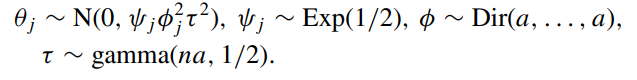# Dirichlet-Laplace prior for shrinkage

Hi,

I’m trying to fit a Bayesian quantile regression model with shrinkage following “Dirichlet–Laplace Priors for Optimal Shrinkage” (Bhattacharya et al. 2016):I’m using K instead of n to denote the number of predictors, and assuming a = 1/K so that \tau is Gamma(1,1/2). Code is below:

def BQR_DL(tau=0.5, a=5, b=.04, X=None, y=None):

T, K = X.shape

# Deterministic
theta            = (1-2*tau)/(tau*(1-tau))
tau_star_squared = 2/(tau*(1-tau))

# Non-Beta Priors
sigma = numpyro.sample('sigma', dist.InverseGamma(a,1/b))

# Beta
phi            = numpyro.sample('phi', dist.Gamma(1,0.5))
v              = numpyro.sample('v', dist.Dirichlet((1/K)*jnp.ones(K)))
psi            = numpyro.sample('psi', dist.Exponential(0.5*jnp.ones(K)))
unscaled_beta  = numpyro.sample("unscaled_beta", dist.Normal(0.0, jnp.ones(K)))
beta           = numpyro.deterministic("beta",  jnp.sqrt(psi*(v**2)*(phi**2)) * unscaled_beta)
beta0          = numpyro.sample('beta0', dist.Normal(0, 5))

z         = numpyro.sample('z', dist.Exponential(1/sigma*jnp.ones(T)))
y_mean    = beta0+jnp.matmul(X,beta)+theta*z
sigma_obs = jnp.sqrt(tau_star_squared*sigma*z)
y         = numpyro.sample("y", dist.Normal(y_mean, sigma_obs),obs=y)

m_DL = MCMC(NUTS(BQR_DL), num_warmup=5000, num_samples=10000, num_chains=1)
m_DL.run(random.PRNGKey(0),X=X,y=y)
m_DL.print_summary(0.89,exclude_deterministic=False)


I’m posting because I’ve estimated 5 other models like this where all that is different is the part of the model that belongs to the variance of beta, which here is:

 # Beta
phi            = numpyro.sample('phi', dist.Gamma(1,0.5))
v              = numpyro.sample('v', dist.Dirichlet((1/K)*jnp.ones(K)))
psi            = numpyro.sample('psi', dist.Exponential(0.5*jnp.ones(K)))
unscaled_beta  = numpyro.sample("unscaled_beta", dist.Normal(0.0, jnp.ones(K)))
beta           = numpyro.deterministic("beta",  jnp.sqrt(psi*(v**2)*(phi**2)) * unscaled_beta)


and of the six, this DL prior one is taking a very, very long time to estimate and returning a signficant number of divergences.

I was just wondering if there is anything obviously wrong with my model that I’m just not seeing? Thanks in advance.

Figured it out. Just needed:

phi            = numpyro.sample('phi', dist.Gamma(K*alpha,0.5))
v              = numpyro.sample('v', dist.Dirichlet(alpha*jnp.ones(K)))


with alpha=0.5, which is the other prior suggested in the paper.

3 Likes